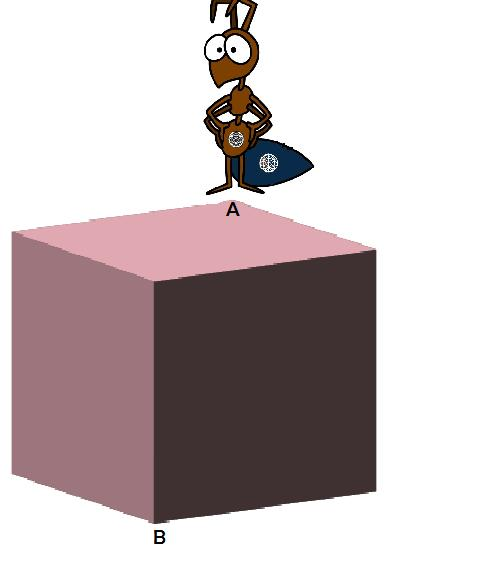# Don't Think Outside The Box!

Geometry Level 2Brilli the ant is trapped on a cube-shaped planet. She wants to go from one corner [point$A$] to the opposite corner [point $B$]. However she wants do this in a way such that she has to cross the shortest distance possible. If the length of the sides of the cube is $1$, the shortest distance between $A$ and $B$ can be expressed as $p+\sqrt{q}$ where $p$ and $q$ are non-negative integers and $q$ is square-free. What is $q-p$?

Details and assumptions:

Brilli the ant is completely confined to the surface of the cube. She can't move inside or outside the cube.

×

Problem Loading...

Note Loading...

Set Loading...